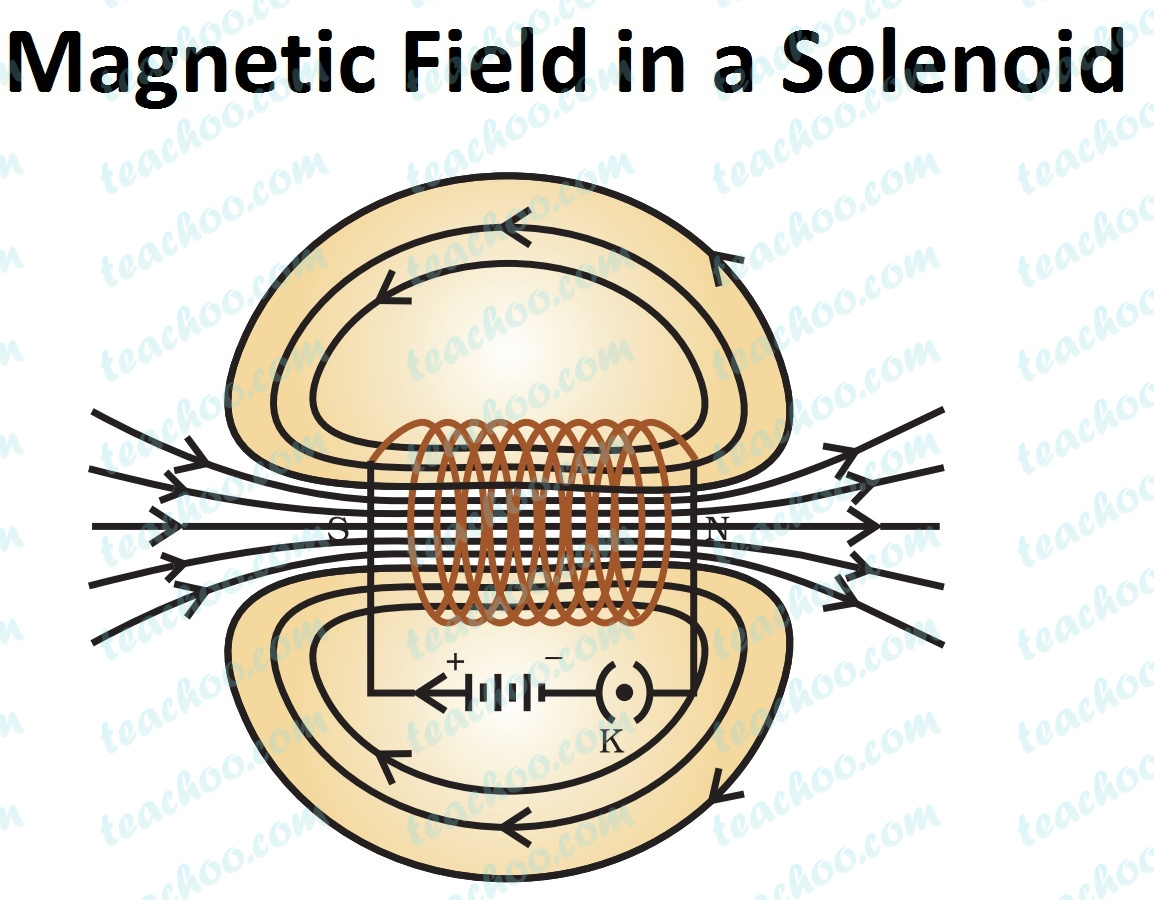Questions from Inside the chapter

Class 10
Chapter 13 Class 10 - Magnetic Effects of Electric Current (Term 2)

## The magnetic field inside a long straight solenoid-carrying current

1. is zero.
2. decreases as we move towards its end.
3. increases as we move towards its end.
4. is the same at all points.

is the same at all points.

Explanation -  Inside a solenoid, Field lines are parallel straight lines

It means that magnetic field is same at all points inside the solenoid